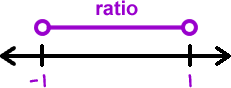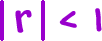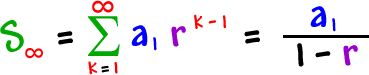OK, now for the mind-blowing infinite thing!

Now, we want to find this sum:And...  we WILL get a finite number for the answer!

Here's the formula...

For this to work, the ratio has got to be greater than -1
and less than 1...(The ratio must be between -1 and 1.)

 The math way to say this is.

So...

 If, thenThat's it!